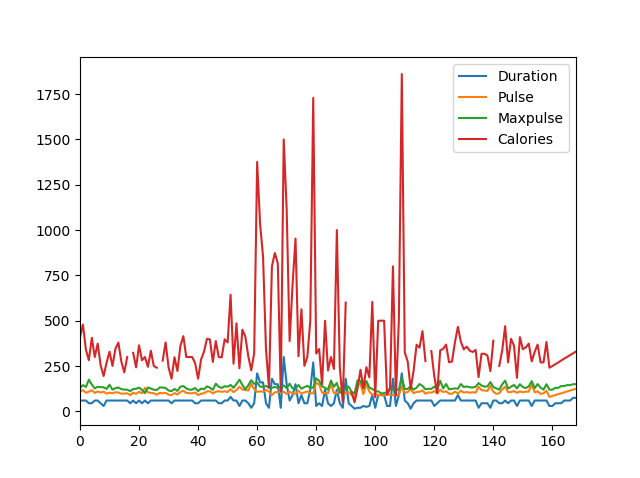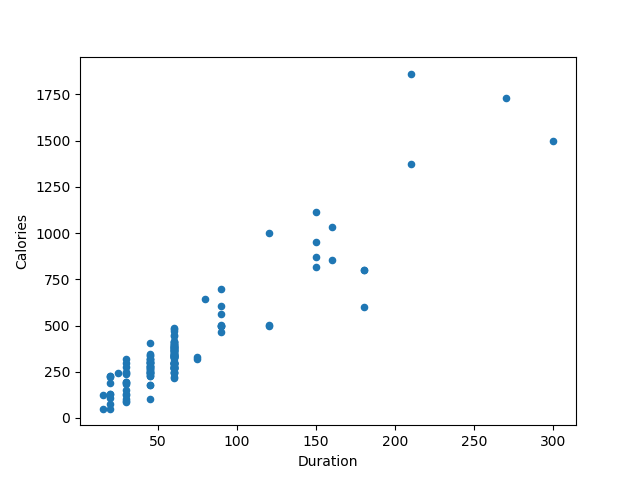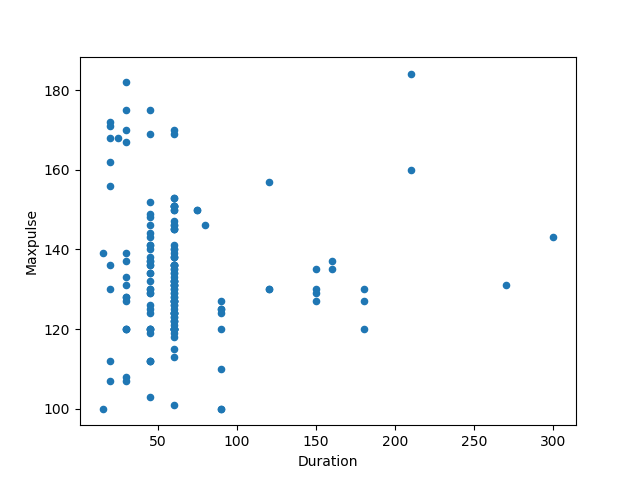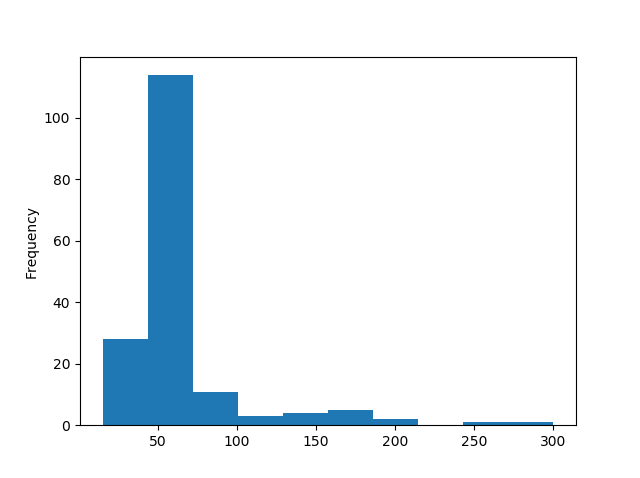## Pandas Tutorial

Pandas HOME Pandas Intro Pandas Getting Started Pandas Series Pandas DataFrames Pandas Read CSV Pandas Read JSON Pandas Analyzing Data

## Cleaning Data

Cleaning Data Cleaning Empty Cells Cleaning Wrong Format Cleaning Wrong Data Removing Duplicates

## Correlations

Pandas Correlations

Pandas Plotting

## Quiz/Exercises

Pandas Editor Pandas Quiz Pandas Exercises Pandas Bootcamp Pandas Certificate

## References

DataFrames Reference

# Pandas - Plotting## Plotting

Pandas uses the `plot()` method to create diagrams.

We can use Pyplot, a submodule of the Matplotlib library to visualize the diagram on the screen.

### Example

Import pyplot from Matplotlib and visualize our DataFrame:

import pandas as pd
import matplotlib.pyplot as plt

df.plot()

plt.show()
Try it Yourself »

## Scatter Plot

Specify that you want a scatter plot with the ``` kind``` argument:

`kind = 'scatter'`

A scatter plot needs an x- and a y-axis.

In the example below we will use "Duration" for the x-axis and "Calories" for the y-axis.

Include the x and y arguments like this:

`x = 'Duration', y = 'Calories'`

### Example

import pandas as pd
import matplotlib.pyplot as plt

df.plot(kind = 'scatter', x = 'Duration', y = 'Calories')

plt.show()

### ResultTry it Yourself »

Remember: In the previous example, we learned that the correlation between "Duration" and "Calories" was `0.922721`, and we concluded with the fact that higher duration means more calories burned.

By looking at the scatterplot, I will agree.

Let's create another scatterplot, where there is a bad relationship between the columns, like "Duration" and "Maxpulse", with the correlation `0.009403`:

### Example

A scatterplot where there are no relationship between the columns:

import pandas as pd
import matplotlib.pyplot as plt

df.plot(kind = 'scatter', x = 'Duration', y = 'Maxpulse')

plt.show()

### ResultTry it Yourself »

## Get Certified!

Complete the Pandas modules, do the exercises, take the exam, and you will become w3schools certified!

\$10 ENROLL

## Histogram

Use the ``` kind``` argument to specify that you want a histogram:

`kind = 'hist'`

A histogram needs only one column.

A histogram shows us the frequency of each interval, e.g. how many workouts lasted between 50 and 60 minutes?

In the example below we will use the "Duration" column to create the histogram:

### Example

df["Duration"].plot(kind = 'hist')

### ResultTry it Yourself »

Note: The histogram tells us that there were over 100 workouts that lasted between 50 and 60 minutes.

## Exercise:

Insert a correct syntax for visualize the data in DataFrame as a diagram (plotting).

```df.()
```

Start the Exercise